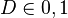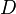# Bayesian Network

## Definition

Credit Scorecards based on Bayesian Network Models (Also, Tree Augmented Naive Bayes Classifier)

This entry serves as the Abstract Risk Model specification of a Bayesian Network Scorecard

## Model Context

A population of borrowers characterised by individual features (characteristics, attributes) associated with each obligor and assumed to represent credit score factors, that is, indicators of propensity to default. The population is modelled statistically for the likelihood for defaulting (or not) over a defined period of time (the Risk Horizon).

## Model Classification

The model belongs to the following categories

• generative (the population characteristics are connected in a graph)
• parametric (a set of weights represents the parameters of the model)
• exclusively observed variables
• (generalized) linear
• supervised (the historical default behaviour represents the label)
• elementary algorithm
• frequentist approach

## Model Description

### Response Variable

The response variable$D \in {0, 1}$ follows a Bernulli distribution. The response variable$D$ captures the realization or not of a Credit Event involving the i-th borrower:

### Explanatory Variables

The Explanatory Variables form an directed acyclical graph (DAG)

### Model Parameters

• Network Structure
• Parameter Weights

### Model Estimation

Markov Chain Monte Carlo